Definitions of Square Dance Calls and Concepts

Index -->  Plus  |  A1  |  A2  |  C1  |  C2  |  C3A  |  C3B  |  C4  |  NOL  |
Definitions (Text Only) -->  Plus  |  A1  |  A2  |  C1  |  C2  |  C3A  |  C3B  |  C4  |  NOL  |
 Find call:

 \$B8@8l(B

Single is a way to take an 8-dancer call and make it a 4-dancer call; or to take a 4-dancer call and make it a 2-dancer call. EN: 10
Single \$B\$O(B, 8 \$B?M\$N%3!<%k\$r(B 4 \$B?M\$N%3!<%k\$K(B, \$B\$"\$k\$\$\$O(B 4 \$B?M\$N%3!<%k\$r(B 2 \$B?M\$N%3!<%k\$K\$9\$kJ}K!\$G\$9!%(B JP: 10

In the Single version of a call, each dancer executes the movement that would normally be done by a set of two dancers within the normal version of the call. Hence, for a call to have a Single version, it must be possible to group all dancers into sets of two, in which the dancers in each set are always facing the same direction thoughout the given call. The dancers in each set are usually dancing their portion of the call As Couples, In Tandem, Once Removed, or Twosome (or some combination of these concepts). EN: 20
\$B\$"\$k%3!<%k\$N(B Single \$B\$G\$O(B, \$B\$=\$N%3!<%k\$N(B 2 \$B?M\$K\$h\$C\$FDL>o9T\$o\$l\$kF0\$-\$r(B, 1 \$B?M\$G9T\$\$\$^\$9!%\$7\$?\$,\$C\$F(B, Single \$B\$,\$"\$k%3!<%k\$O(B, \$BA4\$F\$N?M\$r(B 2 \$B?M\$N=8\$^\$j\$KJ,\$1\$k\$3\$H\$,2DG=\$G(B, \$B\$=\$N(B 2 \$B?M\$O(B, \$B%3!<%k\$rF0\$/4V\$:\$C\$HF1\$8J}\$r8~\$\$\$F\$\$\$kI,MW\$,\$"\$j\$^\$9!%\$=\$N(B 2 \$B?M\$O(B, \$B%3!<%k\$N<+J,\$NItJ,\$r(B As Couples, In Tandem, Once Removed, \$B\$^\$?\$O(B Twosome (\$B\$^\$?\$O\$3\$l\$i\$N%3%s%;%W%H\$NAH\$_9g\$o\$;(B) \$B\$GF0\$-\$^\$9!%(B JP: 20

You can think of Single as replacing each set of two dancers with a single dancer located exactly half-way between the two dancers. This single dancer then executes the given call always staying on a path located exactly half-way between where the two dancers would be in the normal version of the call. EN: 30
Single \$B\$r(B, 2 \$B?M\$N%@%s%5!<\$N=8\$^\$j\$r(B 2 \$B?M\$N%@%s%5!<\$N??\$sCf\$K\$\$\$k(B 1 \$B?M\$N%@%s%5!<\$GCV\$-49\$(\$k(B, \$B\$H9M\$(\$k\$3\$H\$,\$G\$-\$^\$9!%(B \$B\$3\$N(B single \$B\$N%@%s%5!<\$O(B, \$B%3!<%k\$rIaDL\$KF0\$\$\$F\$\$\$k(B 2 \$B?M\$N\$A\$g\$&\$I??\$sCf\$N0LCV\$K\$\$\$D\$b\$\$\$FF0\$/\$3\$H\$K\$J\$j\$^\$9!%(B JP: 30

Here is a partial list of calls that might be done Single at C3B: EN: 40
C3B \$B\$GMY\$i\$l\$k(B Single \$B\$N%3!<%k%j%9%H\$N0lIt\$G\$9(B: JP: 40

 Bounce anyone Checkmate Circle By Cross Chain & Roll Cross Chain Thru Divide The Ocean | Sea Ferris Wheel Flare Out To A Line Polly Wally Rotary Spin | anything Shakedown Shake & Rattle Sidetrack Slant anything Split Swap Strut Left | Right Travel Thru Turn & Deal Turn To A Line Veer Left | Right

In 1996, callers voted to restrict the usage of the Single CONCEPT at C3A to the following calls: EN: 50
1996 \$BG/\$K(B, \$B%3!<%i!<\$NEjI<\$N7k2L(B, C3A \$B\$G\$N(B Single CONCEPT \$B\$N;H\$\$J}\$r(B, \$BJP: 50

In 2000, callers voted to drop the generalized Single CONCEPT from C3B. EN: 60
2000 \$BG/\$K\$O(B, \$B%3!<%i!<\$NEjI<\$N7k2L(B, C3B \$B\$+\$i0lHL2=\$5\$l\$?(B Single CONCEPT \$B\$rMn\$H\$7\$^\$7\$?!%(B JP: 60

Single Ferris Wheel [C3A] (Walt Wentworth 1974):
From a Mini-Wave Box. Leaders do your part Single Wheel, as Trailers Extend & Single Wheel. Ends in a Zero-Tag (Single Starting Double Pass Thru Formation). Equivalent to Follow Thru & Roll. EN: 342
(1 \$B\$D\$NF0\$-\$H\$7\$F\$N(B, Follow Thru & Roll) JP: 342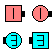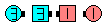Single Ferris Wheel\$B\$NA0(B \$B8e(B

Single Rotary Spin [C3A]:
From a Single Eight Chain Thru. Right Pull By; Centers Step To A L-H Mini-Wave and Cast Off 3/4 as Outsides Left-face U-Turn Back (Single Courtesy Turn) and Roll. Ends in a R-H Wave. EN: 343
Single Eight Chain Thru \$B\$+\$i!%(BRight Pull By \$B\$r\$7\$F(B, Centers \$B\$O(B Step To A L-H Mini-Wave \$B\$r\$7(B, Cast Off 3/4 \$B\$r\$9\$k4V\$K(B, Outsides \$B\$O:82s\$j\$K(B U-Turn Back (Single Courtesy Turn) and Roll \$B\$r\$7\$^\$9!%(BR-H Wave \$B\$G=*\$o\$j\$^\$9!%(B JP: 343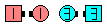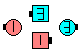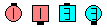Single Rotary Spin\$B\$NA0(B Right Pull By\$B\$r\$7(B \$B\$H(B Centers Step To A L-H Wave\$B\$N8e(B Outsides \$B\$O(B Single Courtesy Turn & Roll as Centers \$B\$O(B Cast Off 3/4\$B\$N8e(B (\$B=*\$o\$j(B)

Single Shakedown [C4] (Lee Kopman 1979):
From Back-to-Back Dancers. As one movement, Right Roll To A Wave & Roll. Ends in Facing Dancers. EN: 345
(1 \$B\$D\$NF0\$-\$H\$7\$F(B, Right Roll To A Wave & Roll) JP: 345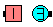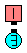Single Shakedown\$B\$NA0(B \$B8e(B

Single Turn & Deal [C4]:
From a Couple or a Mini-Wave. As one movement, 1/4 In, Touch, and turn 1/4 toward your initial turning direction. A Couple ends in Tandem dancers; a R-H Mini-Wave ends in Facing dancers; a L-H Mini-Wave ends in Back-to-Back dancers. From a Couple or R-H Mini-Wave, Single Turn & Deal is the same as Single Wheel [A2]; from a L-H Mini-Wave, Single Turn & Deal ends in Back-to-Back Dancers whereas Single Wheel ends in Facing Dancers. EN: 46
\$B%+%C%W%k\$^\$?\$O(B Mini-Wave \$B\$+\$i!%(B1 \$B\$D\$NF0\$-\$H\$7\$F(B, 1/4 In, Touch \$B\$r\$7(B, \$B;O\$a\$N2sE>\$NJ}\$X(B 1/4 \$B2s\$j\$^\$9!%(BCouple \$B\$+\$i\$O(B Tandem \$B\$G=*\$o\$j(B, R-H Mini-Wave \$B\$+\$i\$O8~\$+\$\$9g\$C\$F=*\$o\$j(B, L-H Mini-Wave \$B\$+\$i\$OGXCf9g\$o\$;\$G=*\$o\$j\$^\$9!%(B JP: 46

R-H Mini-Wave \$B\$+\$i(B: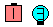Single Turn & Deal\$B\$NA0(B \$B8e(B
L-H Mini-Wave \$B\$+\$i(B: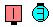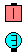Single Turn & Deal\$B\$NA0(B \$B8e(B
Couple \$B\$+\$i(B: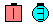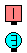Single Turn & Deal\$B\$NA0(B \$B8e(B

\$BCm
• As with Turn & Deal, remember to always pass Right Shoulders! EN: 70
Turn & Deal \$B\$G\$O(B, \$B>o\$K1&8*\$GDL\$k\$3\$H\$r3P\$(\$F\$/\$@\$5\$\$(B! JP: 70
• From a Couple or a R-H Mini-Wave, Single Turn & Deal is identical to Single Wheel [A2]. EN: 80
\$B%+%C%W%k\$^\$?\$O(B R-H Mini-Wave \$B\$+\$i\$N(B Single Turn & Deal \$B\$O(B, Single Wheel [A2] \$B\$HF1\$8\$G\$9!%(B JP: 80
• From a L-H Mini Wave, be sure to pass Right shoulders so that you end Back-to-Back! EN: 90
L-H Mini Wave \$B\$+\$i\$O(B, \$BI,\$:1&8*\$GDL\$j(B, \$BGXCf9g\$o\$;\$K\$J\$j\$^\$9(B! JP: 90

• Single Turn To A Line [C3A]:
From Tandem dancers, Facing dancers, or Back-to-Back dancers. As one movement, Zig-Zag, Step Thru, and turn 1/4 toward your initial turning direction. Ends in a Couple or a Mini-Wave. EN: 344
Tandem \$B\$N(B 2 \$B?M(B, \$B8~\$+\$\$9g\$C\$?(B 2 \$B?M(B, \$B\$^\$?\$OGXCf9g\$o\$;\$N(B 2 \$B?M\$+\$i9T\$\$\$^\$9!%(B1 \$B\$D\$NF0\$-\$H\$7\$F(B, Zig-Zag, Step Thru \$B\$r\$7(B, \$B;O\$a\$N2sE>\$NJ}\$X(B 1/4 \$B2s\$j\$^\$9!%%+%C%W%k\$^\$?\$O(B Mini-Wave \$B\$G=*\$o\$j\$^\$9!%(B JP: 344

Tandem Dancers \$B\$+\$i(B: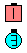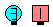Single Turn To A Line\$B\$NA0(B \$B8e(B
Back-to-Back Dancers \$B\$+\$i(B: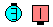Single Turn To A Line\$B\$NA0(B \$B8e(B
Facing Dancers \$B\$+\$i(B: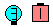Single Turn To A Line\$B\$NA0(B \$B8e(B

There are several calls that contain the word 'Single' but that do not follow the 'Single' definition. For example: EN: 100
'Single' \$B\$N8@MU\$r4^\$`%3!<%k\$,\$\$\$/\$D\$+\$"\$j\$^\$9\$,(B, 'Single' \$B\$NDj5A\$K\$O\$"\$F\$O\$^\$j\$^\$;\$s!%Nc\$(\$P(B: JP: 100Comments? Questions? Suggestions?